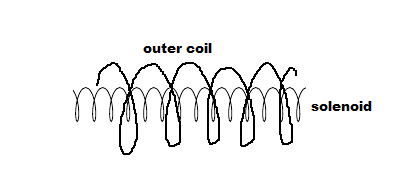# A very long solenoid with a circular cross section and radius r_1 = 1.10 centimeters with n|_s =...

## Question:

A very long solenoid with a circular cross section and radius {eq}r_1 = 1.10 {/eq} centimeters with {eq}n_s = 170 {/eq} turns/ cm lies inside a short coil of radius {eq}r_2 = 4.40 {/eq} centimeters and {eq}N_C = 22 {/eq} turns.If the current in the solenoid is ramped at a constant rate from zero to is {eq}I_s = 1.30 {/eq} Amperes over a time interval of {eq}45.0 {/eq} milliseconds, what is the magnitude of the emf in the outer coil while the current in the solenoid is changing? Note that the {eq}B = \mu_0*n*I {/eq} where "n" s the number of turns per unit length.

## Electromotive Force

The electromotive force is the force that is referred to as the source of energy, and it enables the movement of electron in an electrical circuit. The electromotive force is dependent on the inductance and the rate of change of current.

Given Data

• The radius of the circular cross-section is: {eq}{r_1} = 1.10\;{\rm{cm}} {/eq}
• The number of turns per unit length in solenoid is: {eq}n = 170\;{\rm{turns/cm}} {/eq}
• The radius of the outer coil is: {eq}{r_2} = 4.40\;{\rm{cm}} {/eq}
• The current in solenoid is: {eq}{I_s} = 1.30\;{\rm{A}} {/eq}
• The time is: {eq}t = 45\;{\rm{ms}} {/eq}
• The number of turns in coil is: {eq}{N_C} = 22\;{\rm{turns}} {/eq}

The expression for the cross-sectional area of the solenoid is,

{eq}A = \pi r_1^2 {/eq}

Substitute the known values.

{eq}\begin{align*} A &= \pi {\left( {1.10\;{\rm{cm}}} \right)^2}\\ &= \pi {\left( {0.011\;{\rm{m}}} \right)^2}\\ &= 3.80 \times {10^{ - 4}}\;{{\rm{m}}^2} \end{align*} {/eq}

The expression for the rate of change of the ampere is,

{eq}\dfrac{{dI}}{{dt}} = \dfrac{{{I_s}}}{t} {/eq}

Substitute the known values.

{eq}\begin{align*} \dfrac{{dI}}{{dt}} &= \dfrac{{1.30\;A}}{{45\;{\rm{ms}}}}\\ &= \dfrac{{1.30\;A}}{{45 \times {{10}^{ - 3}}\;{\rm{s}}}}\\ &= 28.888\;{\rm{A/s}} \end{align*} {/eq}

The expression for the mutual inductance is,

{eq}M = {\mu _0}n{N_C}A {/eq}

Substitute the known values.

{eq}\begin{align*} M &= \left( {4\pi \times {{10}^{ - 7}}} \right)\left( {170\;{\rm{turns/cm}}} \right)\left( {22} \right)\left( {3.80 \times {{10}^{ - 4}}} \right)\\ &= \left( {4\pi \times {{10}^{ - 7}}} \right)\left( {17000\;{\rm{turns/m}}} \right)\left( {22} \right)\left( {3.80 \times {{10}^{ - 4}}} \right)\\ &= 1.7859 \times {10^{ - 4}} \end{align*} {/eq}

The expression for the induced emf is,

{eq}emf = M\dfrac{{dI}}{{dt}} {/eq}

Substitute the known values.

{eq}\begin{align*} emf &= 1.7859 \times {10^{ - 4}}\left( {28.888} \right)\\ &= 5.159 \times {10^{ - 3}}\;{\rm{V}}\\ &= 5.159\;{\rm{mV}} \end{align*} {/eq}

Thus, the magnitude of the emf in outer coil is {eq}5.159\;{\rm{mV}} {/eq}.